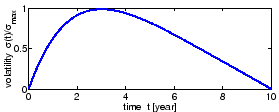SYLLABUS  Previous: 5.1 Discound bonds  Up: 5.1 Discound bonds  Next: 5.1.2 Parameters illustrated with

5.1.1 Term structure models for dummies

[ SLIDE hedging - experiments - forecasting models || VIDEO modem - LAN - DSL ]

Imagine a portfolio with two identical discount bonds, except that the first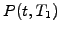expires some time before the second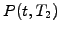. What is the effect of a market fluctuation, which suddenly rises the spot rate at a time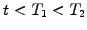before the first bond reaches maturity? The bonds are correlated and both will loose some of their original value; since there is more time left for another fluctuation to step back in the opposite direction, it is reasonable to assume that the second bond with a longer time to maturity will be less affected.

Taking advantage of this correlation, Vasicek creates a portfolio with a positive holding in the first bond and a negative holding in the second. By choosing exactly the right balance, this delta-hedging cancels out the uncertain effect from fluctuations and leaves only a deterministic change in the portfolio value. This is then used to calculate the fair price of a bond. The normalized value of the discount function is of course known at the maturity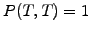and the calculation is carried out with a forecast of the interest rates backward in time to predict the fair value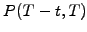for an increasing lifetime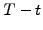.

The VMARKET applet below illustrates the procedure for a bond lifetime with up to RunTime=10 years.

WIDTH="12" HEIGHT="13" ALIGN="BOTTOM" BORDER="0" SRC="s5img48.gif" ALT="$r$"> (horizontal axis, chosen to reflect the current market conditions), the discount function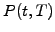is decreasing backward in time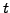. Indeed, investors expect a return from their investment, which shows up as a growth of the discount function when the time runs forward so as to reach exactly one at maturity. The reward can be measured using (2.2.2#eq.1) as a yield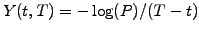and differs from the spot rate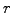because of the uncertain evolution of the future rates.

 Virtual market experiments: evolving the yield curve Press Display to study the evolution of the yield curve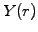for a fixed lifetime of the bond (specified under Time) and the term structure of the interest rates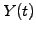that is plotted for the specified SpotRate. Set Volatility=0 and compare the output obtained for a constant interest rate with the simple discounting previously used in (1.3#eq.6).

Due to the cyclic nature of the economy and the changes in the central bank interest rates, economists generally forecast what may be the future evolution of spot rates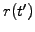with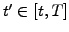. This opinion consists of a drift (the spot rate will fall'') and a volatility (the spot rate will fluctuate'') that can be estimated from historical values (exercise 1.05).

Masters: one factor models to forecast the term structure of interest rates.
A broad class of models can already be obtained using only one driving term for the uncertainty and assuming a normal distribution of the interest rate increments of the form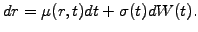(5.1.1#eq.1)

Contrary to stock options where the drift scales out of the Black-Scholes equation (3.4#eq.4), the interest rate drifts play a crucial role for the evolution of bond prices. Using the excess return dP/dt-rP=(-m+ls)dP/dr  when the stochastic term is neglected in (3.5#eq.6), different models have been proposed to forecast the evolution of the interest rates.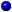The Vasicek model
accounts for a long-term average rate and investors appetite for risk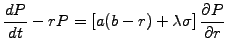(5.1.1#eq.2)

The first term is a mean reversion process, where the interest rate is pulled back to the level b at a velocity a.   The second term is proportional to the market price of risk l   and measures the extra return per unit risk expected by the investors (3.5#eq.9).The Ho and Lee model
uses the instantaneous forward rate F(0,0,t)   from the market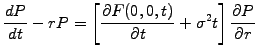(5.1.1#eq.3)

to forecast a drift based on today's expectations without ever saturating.The Hull an White model
circumvents this problem with an evolution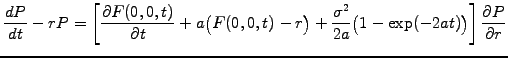(5.1.1#eq.4)

which reproduces the slope of the initial instantaneous forward rates from Ho and Lee, and later revert back to the long-term average F(0,0,t) with a velocity a.The VMARKET model
(c.f. Vasicek) uses a modulation of the market price of risk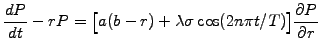(5.1.1#eq.5)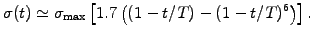(5.1.1#eq.6)